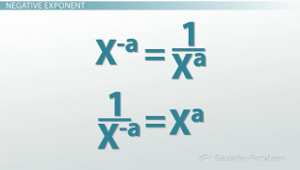# How to Define a Zero and Negative Exponent

An error occurred trying to load this video.

Try refreshing the page, or contact customer support.

Coming up next: How to Simplify Expressions with Exponents

### You're on a roll. Keep up the good work!

Replay
Your next lesson will play in 10 seconds
• 0:06 Negative Exponent
• 1:54 Zero Exponent

Want to watch this again later?

Timeline
Autoplay
Autoplay
Speed

#### Recommended Lessons and Courses for You

Lesson Transcript
Instructor: Kathryn Maloney

Kathryn teaches college math. She holds a master's degree in Learning and Technology.

The zero and negative exponent properties are two you will use quite a lot in mathematics. The negative exponent property can be confusing, but when you remember a couple fun ideas, you will get it right every time!

## Negative ExponentIn math, we like to write exponents with a positive number. So what happens if I get a negative exponent? What about a zero exponent?

Before we get started, I need to tell you something important here: x^-a does not mean -x^a. The negative exponent has nothing to do with positive or negative numbers.

Let's look at the two formulas.

• x^-a = 1/(x^a)
• 1/(x^-a) = x^a

I want to make sure that you understand that x^-a does not mean -x^a. Once again, the negative exponent has nothing to do with positive or negative numbers. If the exponential is negative in the numerator, or the top, it tells us the exponential is actually positive in the denominator. If the exponential is negative in the denominator, or the bottom, it tells us the exponential is actually positive in the numerator.

If you see a negative exponent, flip it to a positive. That is, if the exponent is negative in the numerator, flip it positive to the denominator. If the exponent is negative in the denominator, flip it positive to the numerator.

Let's say I have x^-4. Now remember, x^-4 can be written as a fraction (x^-4)/1. Remember, if it's negative in the numerator, you flip it positive to the denominator. So that's the same thing as saying 1/(x^4).

To unlock this lesson you must be a Study.com Member.

### Register to view this lesson

Are you a student or a teacher?

### Unlock Your Education

#### See for yourself why 30 million people use Study.com

##### Become a Study.com member and start learning now.
Back
What teachers are saying about Study.com

### Earning College Credit

Did you know… We have over 160 college courses that prepare you to earn credit by exam that is accepted by over 1,500 colleges and universities. You can test out of the first two years of college and save thousands off your degree. Anyone can earn credit-by-exam regardless of age or education level.